### Home > CCA2 > Chapter Ch2 > Lesson 2.2.1 > Problem2-95

2-95.

Write a possible equation for each of these graphs. Assume that one mark on each axis is one unit. When you are in class, check your equations on a graphing calculator and compare your results with your teammates. 2-95 HW eTool (Desmos). Homework Help ✎.

 a.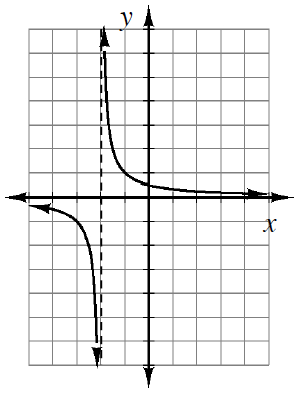Hint (a):Notice that this is a hyperbola $(y=1/x)$. b.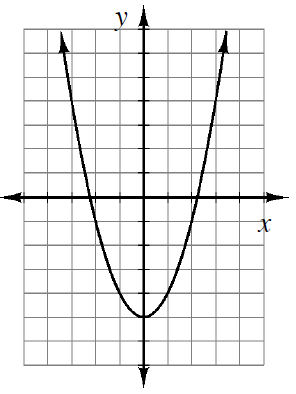Hint (b):Notice that the vertex is (0,−5). c.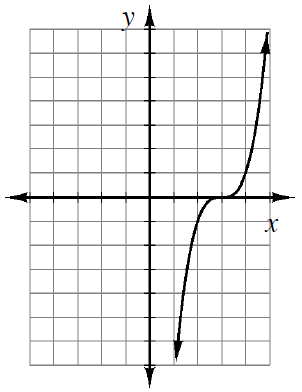Hint (c):Notice that this is a cubic graph.
 d.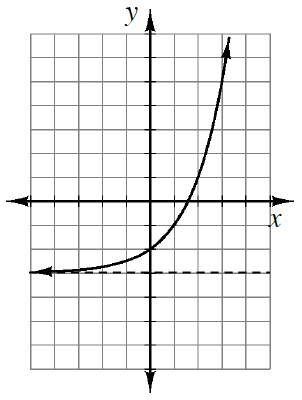Hint (d):Notice that this is an exponential graph. e.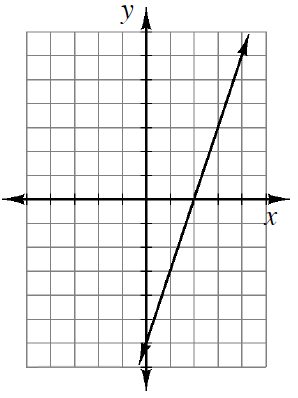Hint (e):Notice that the y-intercept is (0, −6). f.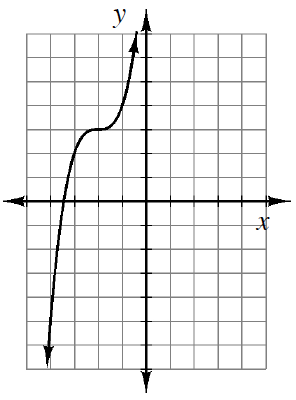Hint (f)-(i):See the hints from parts (a)-(e) to help you solve parts (f)-(i). g.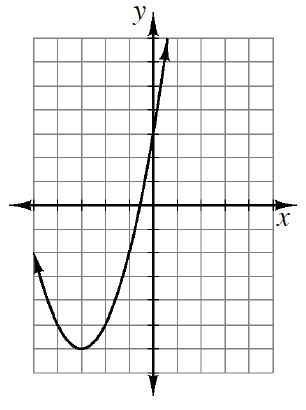h.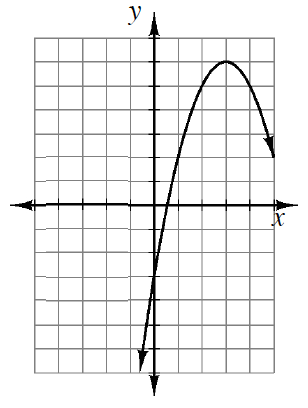i.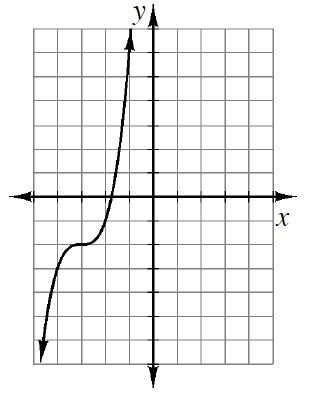Use the eTool below to graph the equations.
Click the link at right for the full version of the eTool: CCA2 2-95 HW eTool